### RSLReflections using trace() & gather()

#### Front ?

The basic code for the RSL listing 1 was taken from Pixar's "Ray-Traced Shading in PRMan" document. If the users computer has Pixar's documentation installed and Cutter's preferences are set correctly the Rman Tools->QLinks can be used to quickly access the document.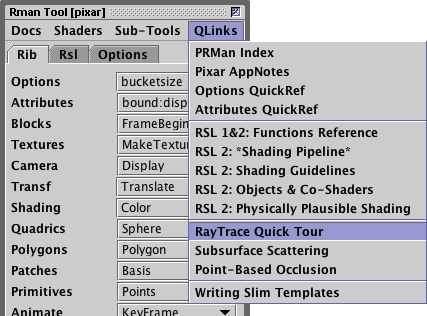Figure 1

The simpletrace.sl code in Pixar's doc implements the test for "front facing" as follows.

```    if(n.i < 0) {
vector r = reflect(i,n);
Ci += trace(P, r);
}```

Figure 2 shows the effect of their shader on a simple scene consisting of two objects (listing 2).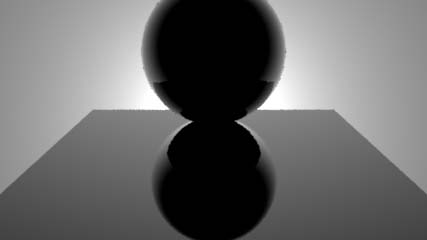Figure 2

##### Beware using a Negative Test
The intention of their "if" test,

`    if(n.i < 0)`

is to ensure the shader only performs a trace from those parts of an object that face the camera ie. front facing surfaces. Yet the test actually asks the question, "is the surface being shaded not a rear face". Even though their "if" test yields the correct results I believe asking, what is in effect, a negative question to be potentially very confusing. Therefore, the sample code (listing 1) uses a slightly modified "if" test. The shader asks the question, "is the surface being shaded a front face"?

#### Basic Code

Listing 1

 ```surface simple_trace() { vector i = normalize(I); normal n = normalize(N), nf = faceforward(n, i); color reflectcolor = 0; /* STEP 1 - assign a default surface color */ Ci = Cs; /* STEP 2 - assign a default surface opacity */ Oi = Os; /* STEP 3 - trace if we are shading a front surface */ if(n.-i >= 0) { vector reflectRay = reflect(i, n); reflectcolor = trace(P, reflectRay); } Ci = Os * reflectcolor; }```Figure 3 Option "trace" "int maxdepth"Figure 4 Option "trace" "int maxdepth" 

Notice in figures 3 and 4 the effect of changing the Option "maxdepth" from 1 to 2. To see the role played by "big_sphere" place a comment in front of its Sphere statement.

Listing 2

 ```Option "trace" "int maxdepth"  Display "shader_tester" "framebuffer" "rgb" Format 427 240 1 Projection "perspective" "fov" 40 ShadingRate 1 LightSource "distantlight" 1 "intensity" 2.5 "from" [0 0 0] "to" [0 0 1] Translate 0 0 5 Rotate -20 1 0 0 Rotate 0 0 1 0 Scale 1 1 -1 WorldBegin Attribute "visibility" "trace"  TransformBegin Attribute "identifier" "name" ["sphere"] Translate 0 1 0 Surface "simple_trace" AttributeBegin Sphere 1 -1 1 360 AttributeEnd TransformEnd TransformBegin Attribute "identifier" "name" ["polygon"] Translate 0 0 1.5 Scale 4 1 4 Surface "simple_trace" Polygon "P" [-0.5 0 -0.5 -0.5 0 0.5 0.5 0 0.5 0.5 0 -0.5] "st" [0 0 0 1 1 1 1 0] TransformEnd TransformBegin Attribute "identifier" "name" ["big_sphere"] Surface "plastic" Color .5 .5 .5 Sphere 100 -100 100 360 TransformEnd WorldEnd```

#### What is trace?

In the snippet of code shown below the function trace determines the color of the light striking a surface at an angle that is equal and opposite to some input angle - generally based on the camera viewing vector.

```    if(n.-i >= 0)
{
vector reflectRay = reflect(i, n);
reflectcolor = trace(P, reflectRay);
}
Ci = Os * reflectcolor;
}```

If instead of using the true angle of reflection we apply a little distortion to the vector returned by the reflect() function we get the bumpy effect shown in figure 5.

```    if(n.-i >= 0)
{
vector reflectRay = reflect(i, n);
reflectRay *= noise(P)/2;
reflectcolor = trace(P, reflectRay);
}
Ci = Os * reflectcolor;
}```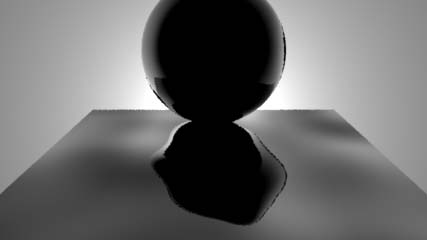Figure 5
Distorting the reflection angle

Figure 6 is a close-up of a highly distorted reflection. Note the aliasing artifacts due to the low sampling of the trace rays. To cure this defect we need a version of the trace() function that "looks" in several directions about a region centered on the angle of reflection.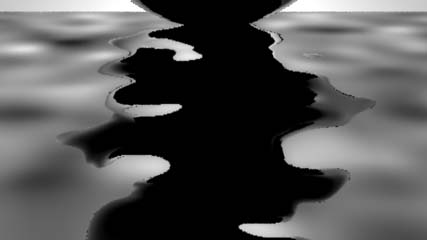Figure 6
Using trace() for extreme distortions

The next function, `gather`() is able to, as its name suggests, gather up a number of rays.

#### What is gather()

The `gather`() function shoots rays out from the surface distributed within a cone centered on the true reflection angle. The parameter samplecone specifies, in radians, the half cone angle. For example, to shoot 12 rays distributed within a cone with a half angle of 0.01 radians would be specified in a rib file as, as,

`    Surface "gather_mirror" "samples" 12 "samplecone" 0.01`

Listing 3

 ```surface gather_mirror (float samples = 1; float samplecone = 0) { color hitc = 0; vector i = normalize(I); normal n = normalize(N), nf = faceforward(n, i); Ci = 0; if(n.-i >= 0) { vector reflectRay = reflect(i, n); reflectRay *= noise(P * 3); float numhits = 0; gather("illuminance", P, reflectRay, samplecone, samples, "surface:Cs", hitc) { Ci += hitc; numhits += 1; } } /* Calculate the average color */ Ci /= numhits; Oi = 1; }```

Its difficult to visualize the cone angle measured in radians but converting radians to degrees (approximately) we have,

0.01 * 2 = 0.02 radians,
or
0.01 * 2 * 57.2958 = 1.15 degrees.

In effect the gather() function performs the `Ci += hitc` calculation each time one of its 12 sample rays hits a surface. The gather rays bounce around in the scene and for each surface they strike they "collect" data of the type specified in the gather() function. In listing 3, the gather rays "collect" the true surface color - Cs. Cs is relatively cheap to gather because it is can be returned without the surface shader on the hit surface being invoked.

```gather("illuminance", P, reflectDirection,
samplecone, samples, "surface:Cs", hitc)
```

For each of the (sample) rays the function stores the result in the variable hitc (aka hit color). Notice the shader takes the average by dividing the accumulated color by the number of hits.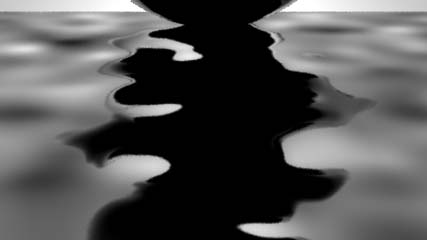Figure 8
Using gather() for extreme distortions